Goseeko blog# Taylor series method

Taylor series method to solve the first order ordinary differential equation– The general first order differential equation

With the initial condition y(x0) = y0 …(2)

In general, the solution of first order differential equation in one of the two forms:

a) A series for y in terms of power of x, from which we can obtain the value of y by direct solution.

b)   A set of tabulated values of x and y.

We solve case (a) by Taylor’s Series or Picard method whereas case (b) by Euler’s, Runge Kutta Methods etc.

Taylor’s Series Method:

The general first order differential  equation

With the initial condition  y(x0) = y0 …(2)

Let  be the exact solution of equation (1), then the Taylor’s series for  around  is given by

If the values of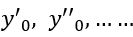are known, then equation (3) gives apowwer series   for y. By total derivatives   we have

And other higher derivatives of y.  The method can easily  be extended to   simultaneous   and higher –order  differential  equations. In   general,

Putting  x = x0 and y = y0 in these above results, we can obtain the values of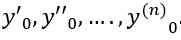.finally, we substitute these values of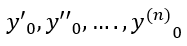in equation (2) and obtain the approximate value of y; i.e. the solutions of (1).

Example1: Solve,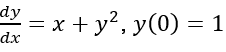using Taylor’s series method and compute y(0.1) and y(0.2).

Sol:

Here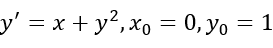This implies that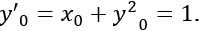Differentiating, we get

The   Taylor’s series at x = x0,

At x = 0.1 in equation (1) we get

At x = 0.2 in equation (1) we get